Win up to 100% scholarship on Aakash BYJU'S JEE/NEET courses with ABNAT Win up to 100% scholarship on Aakash BYJU'S JEE/NEET courses with ABNAT

# Principle of Superposition of Waves

## What Is the Superposition of Waves?

According to the principle of superposition, the resultant displacement of a number of waves in a medium at a particular point is the vector sum of the individual displacements produced by each of the waves at that point.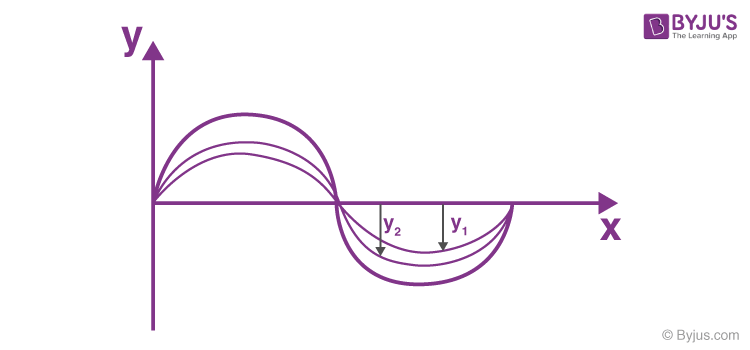## Principle of Superposition of Waves

Consider two waves travelling simultaneously along the same stretched string in opposite directions, as shown in the figure above. We can see images of waveforms in the string at each instant of time. It is observed that the net displacement of any element of the string at a given time is the algebraic sum of the displacements due to each wave.

Let us say two waves are travelling alone, and the displacements of any element of these two waves can be represented by y1(x, t) and y2(x, t). When these two waves overlap, the resultant displacement can be given as y(x,t).

Mathematically, y (x, t) = y1(x, t) + y2(x, t).

As per the principle of superposition, we can add the overlapped waves algebraically to produce a resultant wave. Let us say the wave functions of the moving waves are

y1 = f1(x–vt),

y2 = f2(x–vt)

……….

yn = fn (x–vt)

Then, the wave function describing the disturbance in the medium can be described as,

y = f1(x – vt)+ f2(x – vt)+ …+ fn(x – vt)

or, y=∑ i=1 to n = fi (x−vt)

Let us consider a wave travelling along a stretched string given by, y1(x, t) = A sin (kx – ωt) and another wave, shifted from the first by a phase φ, given as y2(x, t) = A sin (kx – ωt + φ)

From the equations, we can see that both the waves have the same angular frequency, the same angular wave number k, and hence the same wavelength and the same amplitude A.

Now, applying the superposition principle, the resultant wave is the algebraic sum of the two constituent waves and has displacement y(x, t) = A sin (kx – ωt) + A sin (kx – ωt + φ).

The above equation can be written as,

y(x, t) = 2A cos (ϕ/2). sin (kx − ωt + ϕ/2)

The resultant wave is a sinusoidal wave, travelling in the positive X direction, where the phase angle is half of the phase difference of the individual waves and the amplitude as [2cos ϕ/2] times the amplitudes of the original waves.

### Superposition of Waves – Video Lesson## What Is Interference of Light?

The phenomena of formation of maximum intensity at some points and minimum intensity at some other point when two (or) more waves of equal frequency having constant phase difference arrive at a point simultaneously, superimpose with each other, is known as interference.

## Types of Superposition of Waves

According to the phase difference in superimposing waves, interference is divided into two categories as follows.

### Constructive Interference

If two waves superimpose with each other in the same phase, the amplitude of the resultant is equal to the sum of the amplitudes of individual waves resulting in the maximum intensity of light; this is known as constructive interference.

### Destructive Interference

If two waves superimpose with each other in opposite phases, the amplitude of the resultant is equal to the difference in amplitude of individual waves, resulting in the minimum intensity of light; this is known as destructive interference.

### Resultant Intensity in Interference of Two Waves

Let two waves of vertical displacements, y1 and y2, superimpose at a point p in space, as shown in the figure, then the resultant displacement is given by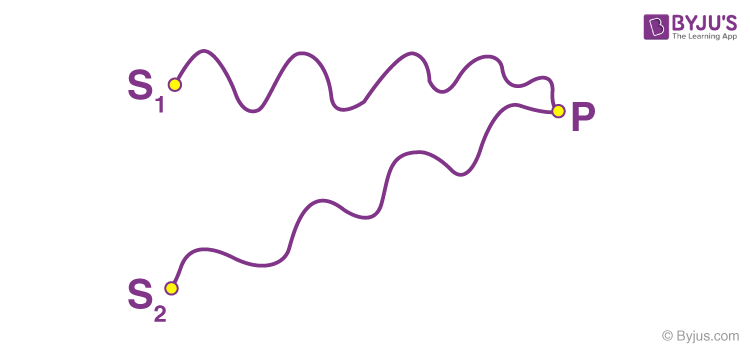y = y1 + y2

Waves meet at some point p, and at the same time, the only difference occurs in their phases. Displacements of individual waves are given by

y1 = a sin ωt

y2 = b sin ( ωt + φ)

Where, a and b are their respective amplitudes, and Φ is the constant phase difference between the two waves.

Applying the superposition principle as stated above, we get

y = a sin ωt + b sin (ωt + θ) . . . . . . . . . . (1)

Representing equation 1 in the phasor diagram,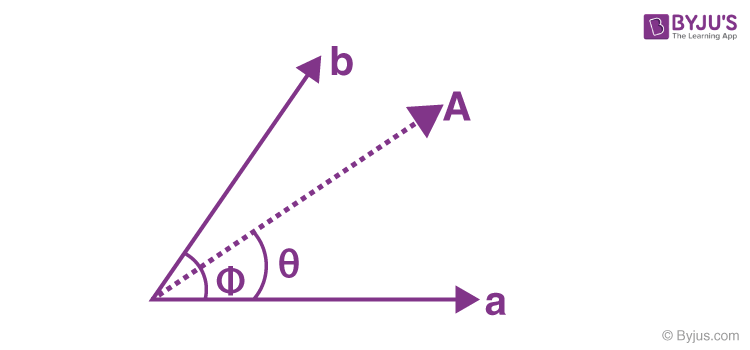The resultant having an amplitude A and a phase angle with respect to wave —1

y = A sin(ωt + θ)

A sin(ωt + θ) = a sin ωt + b sin (ωt + θ)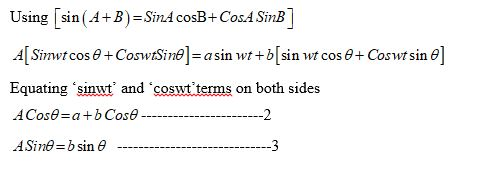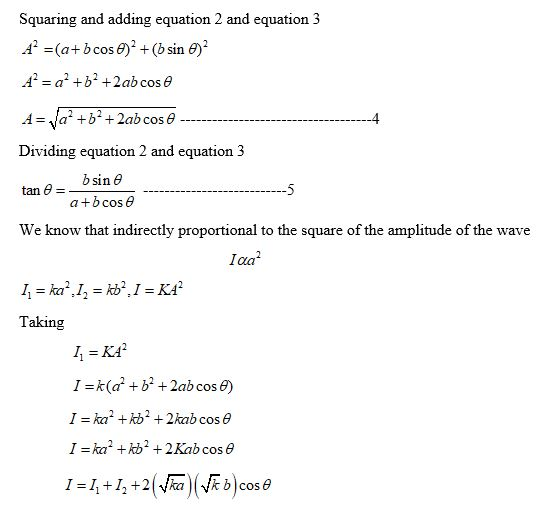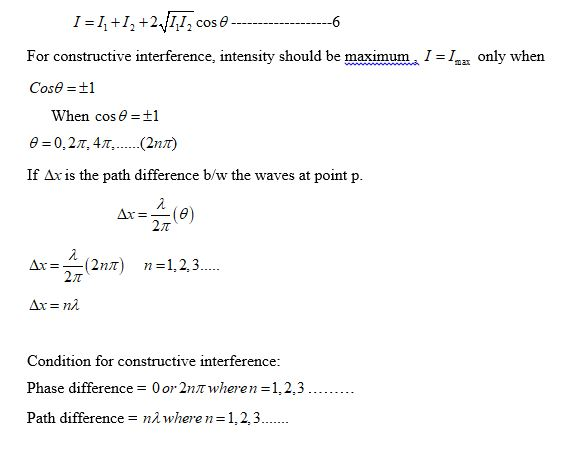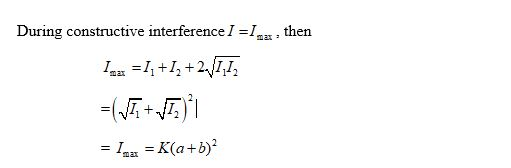For destructive interference, intensity should be minimum I = Imin, which happens only when cos φ = -1

When cos φ = -1

φ = π , 3π, 5π, . . . . . .

⇒ φ = (2n – 1)π, when n = 1, 2, 3, . . . . . .

Of Δx is the path difference between the waves at point p.

$$\begin{array}{l}\Delta x=\frac{\lambda }{2\pi }\phi\end{array}$$
$$\begin{array}{l}\Delta x=\frac{\lambda }{2{\pi }}\left( 2n-1 \right){\pi }\end{array}$$

Therefore,

$$\begin{array}{l}\Delta x=\frac{\left( 2n-1 \right)}{2}\lambda\end{array}$$

### Condition for Destructive Interference

Phase difference = (2n – 1)π

Path difference = (2n – 1)λ/2

I = Imin

$$\begin{array}{l}{{I}_{\min }}={{I}_{1}}+{{I}_{2}}-2\sqrt{{{I}_{1}}{{I}_{2}}}\end{array}$$
$$\begin{array}{l}={{\left( \sqrt{{{I}_{1}}}-\sqrt{{{I}_{2}}} \right)}^{2}}\end{array}$$

### Conditions for Interference of Light

• Sources must be coherent
• Coherent sources must have the same frequency (monochromatic light source)
• The waves from the coherent sources should be of equal amplitudes

## Frequently Asked Questions on Superposition of Waves

Q1

### What happens when the waves superimpose?

Constructive interference and destructive interference.

Q2

### Define nodes and antinodes.

Nodes are points of zero amplitude, and antinodes are points of maximum amplitude.

Q3

### What is constructive interference?

If we add two waves together by superposition, the new wave has a larger amplitude but looks similar to the original waves. This situation, where the resultant wave is bigger than either of the two original waves, is called constructive interference.

Q4

### What is destructive interference?

When the two waves superimpose, the sum of the two waves can be less than either wave and can also be zero. This is called destructive interference.

Test your Knowledge on Superposition of waves